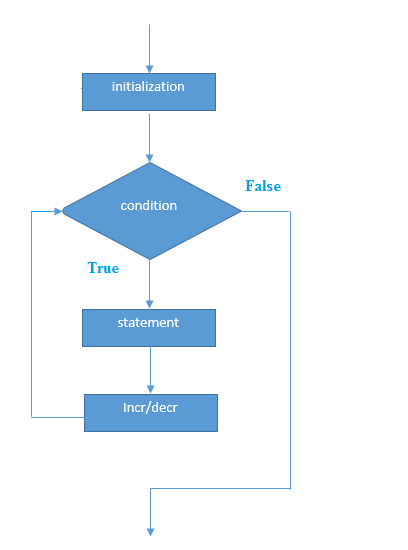# Java Tutorial

## Java While Loop

The test expression inside parenthesis is a boolean expression.

Loop is used in programming to repeat a specific block of code. In this article, you will learn to create while and do...while loops in Java programming.

Loop is used in programming to repeat a specific block of code until certain condition is met (test expression is false).

Loops are what makes computers interesting machines. Imagine you need to print a sentence 50 times on your screen. Well, you can do it by using print statement 50 times (without using loops). How about you need to print a sentence one million times? You need to use loops.

It's just a simple example. You will learn to use while and do..while loop to write some interesting programs in this article.

##### If the test expression is evaluated to true,
• statements inside the while loop are executed.
• then, the test expression is evaluated again.

This process goes on until the test expression is evaluated to false.

If the test expression is evaluated to false,

• while loop is terminated.
##### Syntax:
while(condition) { //code to be executed }
##### Flowchart of while Loop##### Write a program in Java to display n terms of natural numbers
```
public class WhileExample
{
public static void main(String[] args)
{
int i=1;
System.out.println("Display 10 Natural numbers:");
while(i<=10)
{
System.out.println(i);
i++;
}
}
}

```

##### Output:
```
Display 10 Natural numbers:

1
2
3
4
5
6
7
8
9
10
```
##### Write a program in Java to display n terms of natural numbers
```
import java.util.Scanner;
public class WhileExample
{
public static void main(String[] args)
{
int i=1,n;
Scanner p=new Scanner(System.in);
System.out.println("Enter any value:");
n=p.nextInt();
System.out.println("Display "+n+" natural numbers");
while(i<=n)
{
System.out.println(i);
i++;
}
}
}
```

##### Output:
```
Enter any value:
20
Display 20 natural numbers
1
2
3
4
5
6
7
8
9
10
11
12
13
14
15
16
17
18
19
20

```
##### Write a program in Java to display the n terms of even natural number
```
import java.util.Scanner;
public class WhileExample
{
public static void main(String[] args)
{
int i=1,n;
Scanner p=new Scanner(System.in);
System.out.println("Enter any value:");
n=p.nextInt();
System.out.println("Display "+n+" natural numbers");
while(i<=n)
{
if(i%2==0)
{
System.out.println(i);
}
i++;
}
}
}
```

##### Output:
```
Enter any value:
30
Display 30 natural numbers
2
4
6
8
10
12
14
16
18
20
22
24
26
28
30
```
##### Java Infinitive While Loop

If you pass true in the while loop, it will be infinitive while loop.

##### Syntax:
```
while(true)
{
//code to be executed
}
```
```
public class WhileExample2
{
public static void main(String[] args)
{
while(true)
{
System.out.println("infinitive while loop");
}
}
}

```

##### Output:
```
infinitive while loop
infinitive while loop
infinitive while loop
infinitive while loop
infinitive while loop
ctrl+c
```